2018-07-13 22:56:29

upd1:更新5.2.2-对于该子目所阐述的操作“用两个堆来维护一些查询第k小/大的操作”更新了一道例题-该操作对于中位数题目的求解

upd2：更新5.3-利用堆来维护可以“反悔的贪心”

continue...

# 一.堆的性质

1.堆是一颗完全二叉树

2.堆的顶端一定是“最大”，最小”的，但是要注意一个点，这里的大和小并不是传统意义下的大和小，它是相对于优先级而言的，当然你也可以把优先级定为传统意义下的大小，但一定要牢记这一点，初学者容易把堆的“大小”直接定义为传统意义下的大小，某些题就不是按数字的大小为优先级来进行堆的操作的

（但是为了讲解方便，下文直接把堆的优先级定为传统意义下的大小，所以上面跟没讲有什么区别？

3.堆一般有两种样子，小根堆和大根堆，分别对应第二个性质中的“堆顶最大”“堆顶最小”，对于大根堆而言，任何一个非根节点，它的优先级都小于堆顶，对于小根堆而言，任何一个非根节点，它的优先级都大于堆顶（这里的根就是堆顶啦qwq）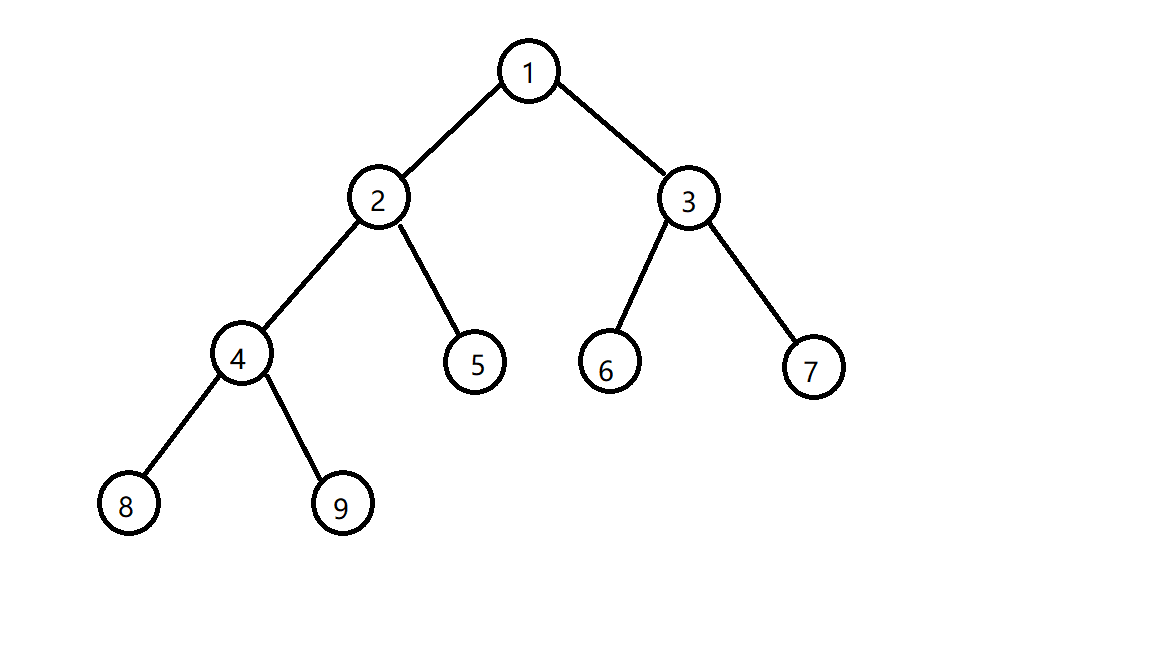# 二.堆的操作

## 1.插入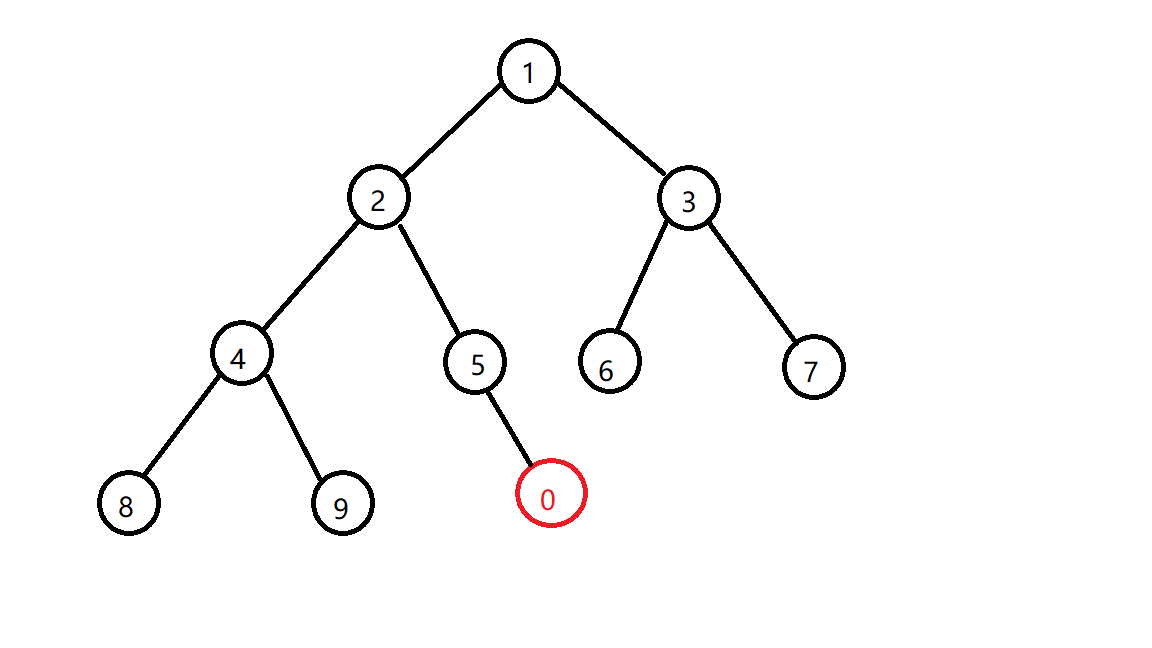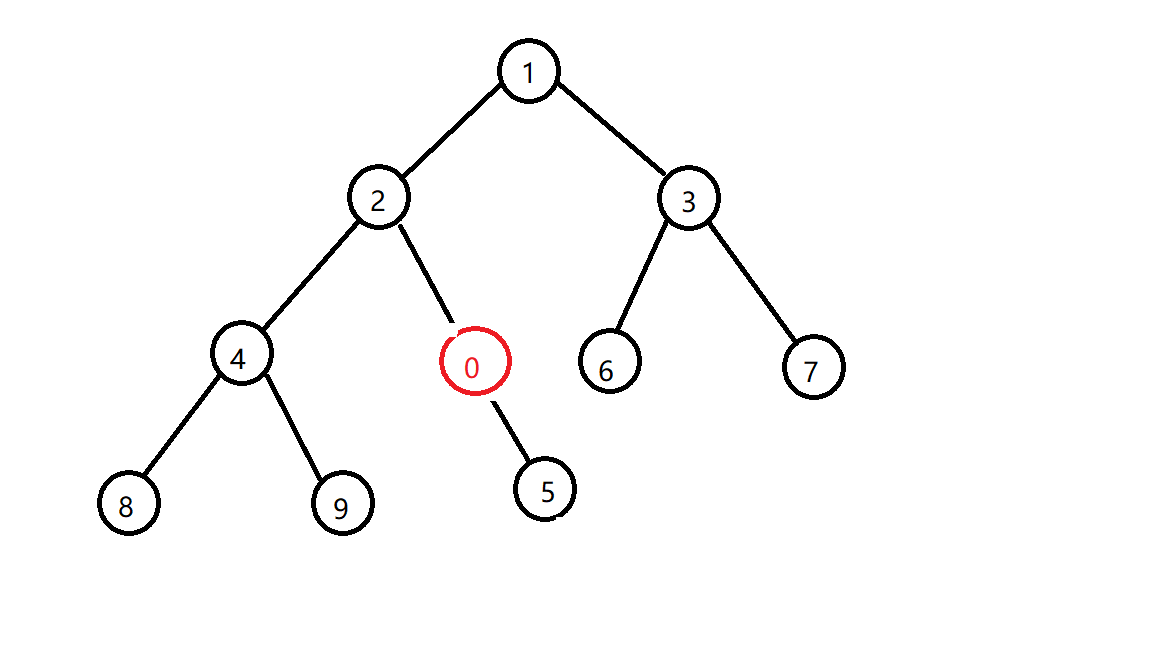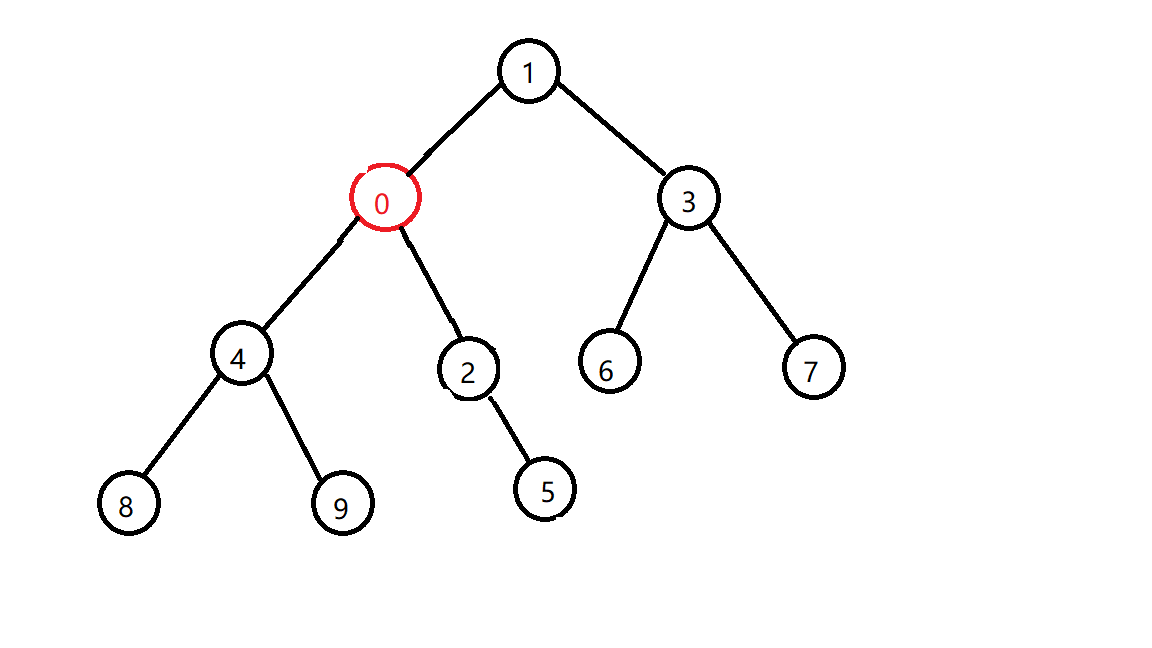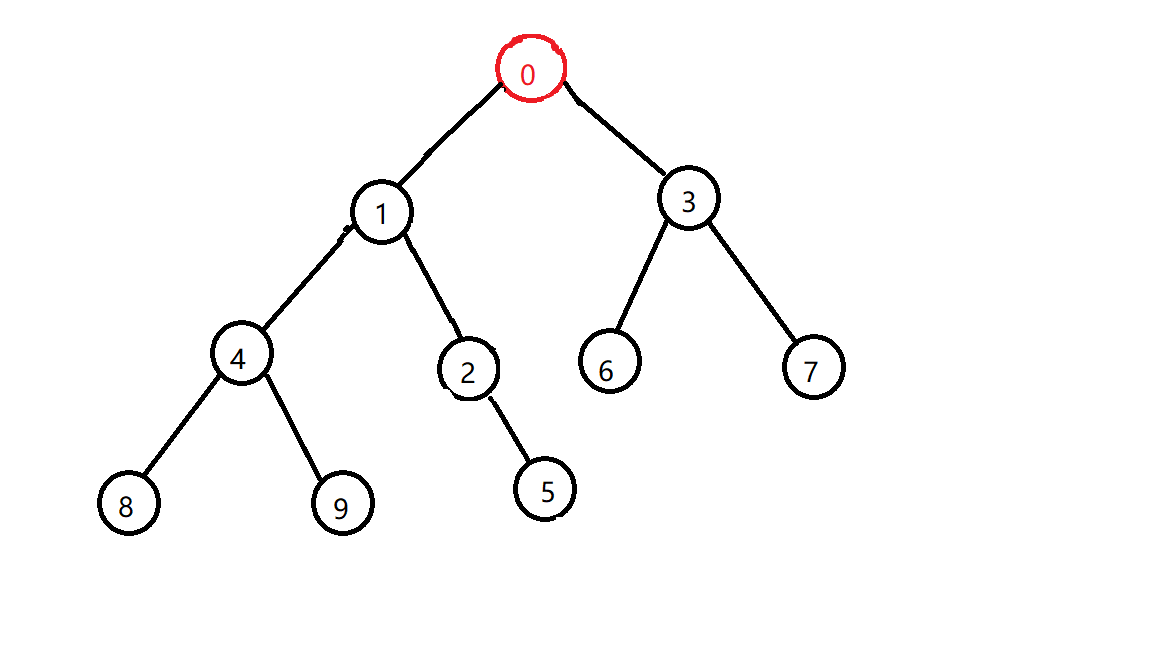Code：

void swap(int &x,int &y){int t=x;x=y;y=t;}//交换函数
int heap[N];//定义一个数组来存堆
int siz;//堆的大小
void push(int x){//要插入的数
heap[++siz]=x;
now=siz;
//插入到堆底
while(now){//还没到根节点，还能交换
ll nxt=now>>1;//找到它的父亲
if(heap[nxt]>heap[now])swap(heap[nxt],heap[now]);//父亲比它大，那就交换
else break;//如果比它父亲小，那就代表着插入完成了
now=nxt;//交换
}
return;
}

## 2.删除Code：

void pop(){
swap(heap[siz],heap);siz--;//交换堆顶和堆底，然后直接弹掉堆底
int now=1;
while((now<<1)<=siz){//对该节点进行向下交换的操作
int nxt=now<<1;//找出当前节点的左儿子
if(nxt+1<=siz&&heap[nxt+1]<heap[nxt])nxt++;//看看是要左儿子还是右儿子跟它换
if(heap[nxt]<heap[now])swap(heap[now],heap[nxt]);//如果不符合堆性质就换
else break;//否则就完成了
now=nxt;//往下一层继续向下交换
}
}

# 三.堆的STL实现

首先你需要一个头文件：#include<queue>
priority_queue<int> q;//这是一个大根堆q
priority_queue<int,vector<int>,greater<int> >q;//这是一个小根堆q
//注意某些编译器在定义一个小根堆的时候greater<int>和后面的>要隔一个空格，不然会被编译器识别成位运算符号>>

q.top()//取得堆顶元素，并不会弹出
q.pop()//弹出堆顶元素
q.push()//往堆里面插入一个元素
q.empty()//查询堆是否为空，为空则返回1否则返回0
q.size()//查询堆内元素数量

struct node{
int val,rnd;
}a;

struct node{
int val,rnd;
bool operator < (const node&x) const {
return rnd<x.rnd;
}
}a;

# 五.堆的应用

## 2.用两个堆来维护一些查询第k小/大的操作

Luogu中也有此类例题，题解内也讲的比较清楚了，此处不再赘述，读者可当做拓展练习进行食用

#include <cstdio>
#include <vector>
#include <cstring>
#include <queue>
#define ll long long
#define inf 1<<30
#define il inline
#define in1(a) read(a)
#define in2(a,b) in1(a),in1(b)
#define in3(a,b,c) in2(a,b),in1(c)
#define in4(a,b,c,d) in2(a,b),in2(c,d)
il int max(int x,int y){return x>y?x:y;}
il int min(int x,int y){return x<y?x:y;}
il int abs(int x){return x>0?x:-x;}
il void swap(int &x,int &y){int t=x;x=y;y=t;}
il void readl(ll &x){
x=0;ll f=1;char c=getchar();
while(c<'0'||c>'9'){if(c=='-')f=-f;c=getchar();}
while(c>='0'&&c<='9'){x=x*10+c-'0';c=getchar();}
x*=f;
}
il void read(int &x){
x=0;int f=1;char c=getchar();
while(c<'0'||c>'9'){if(c=='-')f=-f;c=getchar();}
while(c>='0'&&c<='9'){x=x*10+c-'0';c=getchar();}
x*=f;
}
using namespace std;
/*===================Header Template=====================*/
#define N 200010
priority_queue<int,vector<int>,greater<int> > q;
priority_queue<int> q1;
int n,m,a[N],b[N];
int main(){
in2(n,m);
for(int i=1;i<=n;i++)in1(a[i]);
for(int i=1;i<=m;i++)in1(b[i]);
int i=1;
for(int j=1;j<=m;j++){
for(;i<=b[j];i++){
q1.push(a[i]);
if(q1.size()==j)q.push(q1.top()),q1.pop();
}
printf("%d\n",q.top());
q1.push(q.top());q.pop();
}
return 0;
}

## 3.利用堆来维护可以“反悔的贪心”

#include <cstdio>
#include <algorithm>
#include <queue>
#include <vector>
#include <map>
#include <set>

using namespace std ;

#define N 100010
#define int long long

int n , m ;
struct node {
int d,p ;
bool operator < ( const node &x ) const { return p>x.p; }
} a[ N ] ;

bool cmp( node a , node b ) {
return a.d==b.d?a.p>b.p:a.d<b.d;
}

priority_queue< node > q ;

signed main() {
scanf( "%lld" , &n ) ;
for( int i = 1 ; i <= n ; i ++ ) {
scanf( "%lld%lld" , &a[i].d , &a[i].p ) ;
}
sort(a+1,a+n+1,cmp);
int ans = 0 ;
for( int i = 1 ; i <= n ; i ++ ) {
if( a[i].d<=(int)q.size() ) {
if( q.top().p<a[i].p ) {
ans += a[i].p-q.top().p ;
q.pop() ;
q.push(a[i]) ;
}
} else q.push(a[i]) , ans += a[ i ].p ;
}
printf( "%lld\n" , ans ) ;
}

continue...持续更新中

• star
首页